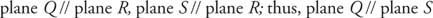## Parallel and Perpendicular Planes

You may be tempted to think of planes as vehicles to be found up in the sky or at the airport. Well, rest assured, geometry is no fly‐by‐night operation.

## Parallel planes

Parallel planes are two planes that do not intersect. In Figure 1, plane P // plane Q.Figure 1 Parallel planes

Theorem 11: If each of two planes is parallel to a third plane, then the two planes are parallel to each other (Figure 2).Figure 2 Two planes parallel to a third plane## Perpendicular planes

A line l is perpendicular to plane A if l is perpendicular to all of the lines in plane A that intersect l. (Think of a stick standing straight up on a level surface. The stick is perpendicular to all of the lines drawn on the table that pass through the point where the stick is standing).

A plane B is perpendicular to a plane A if plane B contains a line that is perpendicular to plane A. (Think of a book balanced upright on a level surface.) See Figure 3.Figure 3 Perpendicular planes

Theorem 12: If two planes are perpendicular to the same plane, then the two planes either intersect or are parallel.

In Figure 4, plane B ⊥ plane A, plane C ⊥ plane A, and plane B and plane C intersect along line l.Figure 4 Two intersecting planes that are perpendicular to the same plane

In Figure 5, plane B ⊥ plane A, plane C ⊥ plane A, and plane B // plane C.Figure 5 Two parallel planes that are perpendicular to the same plane